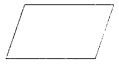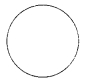• circle
• rectangle
• square
• triangle
• #### 2.  The diagram has the shape of a• rectangle
• square
• parallelogram
• circle
• #### 3.  The diagram has the shape of a• circle
• square
• rectangle
• parallelogram
• #### 4.  The diagram has the shape of a• circle
• parallelogram
• rectangle
• trapezium

• 7 cm
• 14 cm
• 12 cm
• 24 cm

• 8 cm²
• 6 cm²
• 12 cm²
• 16 cm²

• 5 cm
• 10 cm
• 4 cm
• 8 cm

• 6 cm²
• 12 cm²
• 5 cm²
• 10 cm²

• 12 cm
• 24 cm
• 6 cm
• 60 cm
• #### 10.  The area of the figure is• 9 cm²
• 18 cm²
• 12 cm²
• 15 cm²
Report Question
Practice More On Mensuration
access_time